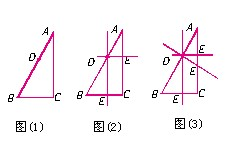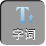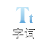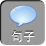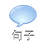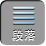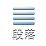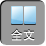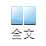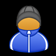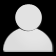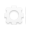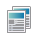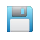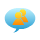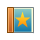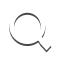-AA+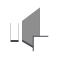1、已知线段 AB= 2 mmCD= 6 cm，则 ABCD=．

ABCD= 26= 13

ABCD= 260= 130

2、如图，在四边形 ABCD与四边形 EFGH中，∠ A= 80°，∠ B= 90°，∠ C= 120°，∠ F= 90°，∠ G= 120°，∠ H= 70°，四边形 ABCD与四边形 EFGH相似吗？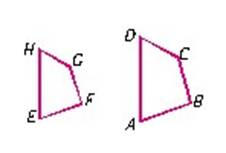∴∠ A=∠ E，∠ B=∠ F，∠ C=∠ G，∠ D=∠ H

∴四边形 ABCD与四边形 EFGH相似．

∴∠ A=∠ E，∠ B=∠ F，∠ C=∠ G

D=∠ H，但是根据已知条件无法判定对应边是否成比例．

∴四边形 ABCD与四边形 EFGH不一定相似．

3、如图，在△ ABC中， DEBC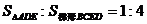，求 ADDB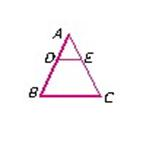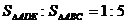2）有时错认为在由面积求相似比时，不开方反而平方；

3）不相似的图形也用了相似的性质进行推导．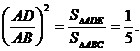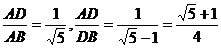4、在△ ABC和△ ABC′中，∠ A=∠ A′= 45°，∠ B= 26°，∠ B′= 109°，它们是否相似？

∴∠ C= 180° -A -B= 109°．

∴∠ C=∠ B′．

∴△ ABC∽△ ACB′．

5、如图（ 1），已知∠ C= 90°， DAB上一点，在 ACBC上找一点 E，使新形成的三角形与 RtABC相似，则满足条件的点 E有几个？